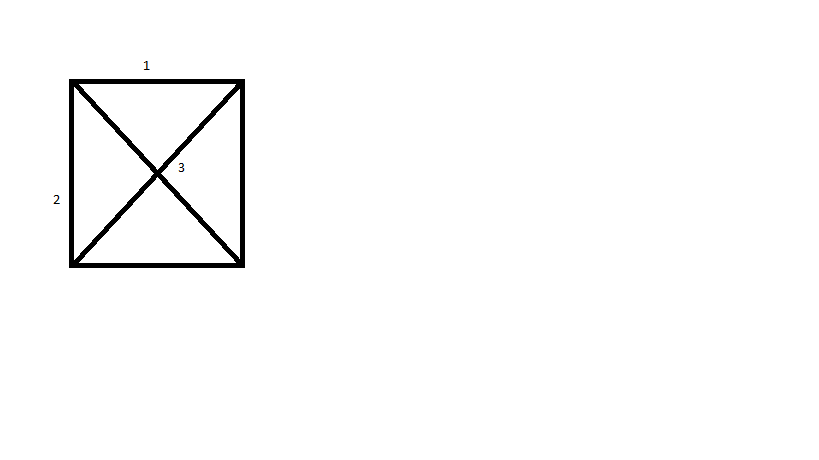##### What is the maximum possible weight that a minimum weight spanning tree of G can have ?

Let G be a complete undirected graph on 4 vertices, having 6 edges with weights being 1, 2, 3,  4, 5, and 6. The maximum possible weight that a minimum weight spanning tree of G can have is __________if we place 3 on diagonal it will form a cycle so it is not taken , else if it is placed somewhere else then MST=1+2+3=6